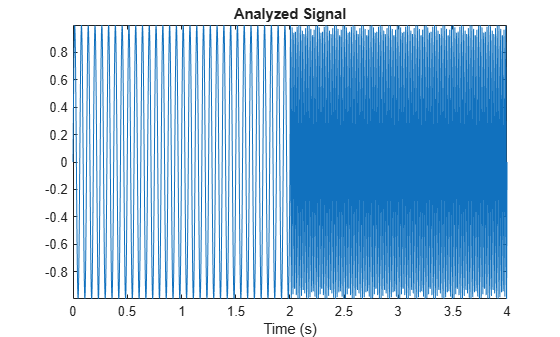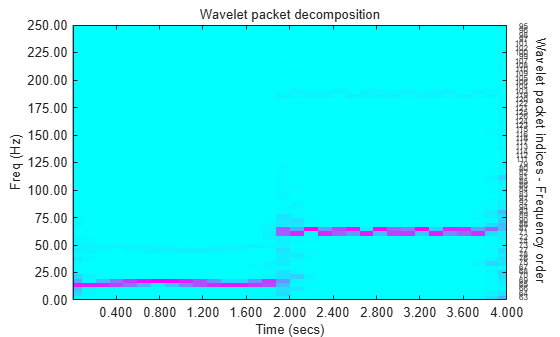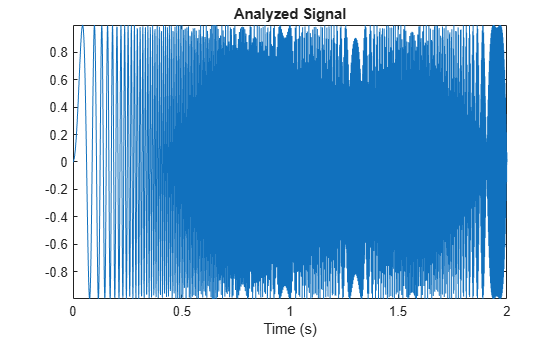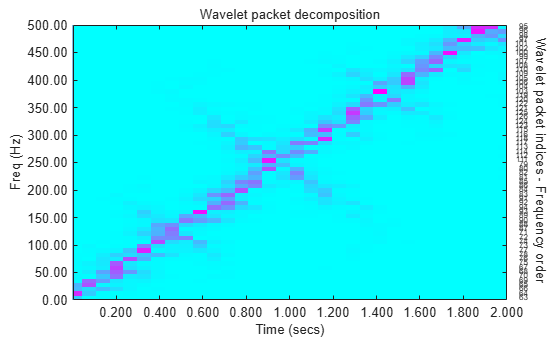# wpspectrum

Wavelet packet spectrum

## Syntax

``[spec,times,freq] = wpspectrum(wpt,fs)``
``[___] = wpspectrum(wpt,fs,'plot')``
``[___,tinfo] = wpspectrum(___)``

## Description

````[spec,times,freq] = wpspectrum(wpt,fs)` returns a matrix of wavelet packet spectrum estimates, `spec`, for the binary wavelet packet tree object, `wpt`. `fs` is the sampling frequency in hertz. `times` is a vector of times and `freq` is a vector of frequencies.```

example

````[___] = wpspectrum(wpt,fs,'plot')` displays the wavelet packet spectrum.```
````[___,tinfo] = wpspectrum(___)` returns the terminal nodes of the wavelet packet tree in frequency order.```

## Examples

collapse all

Create a signal consisting of two sinusoids with disjoint support. The sinusoids have frequencies of 16 Hz and 64 Hz. Sample the signal at 500 Hz for 4 seconds.

```fs = 500; frA = 16; frB = 64; t = 0:1/fs:4; sig = sin(frA*2*pi*t).*(t<2) + sin(frB*2*pi*t).*(t>=2); plot(t,sig) axis tight title('Analyzed Signal') xlabel('Time (s)')```Obtain the wavelet packet tree object corresponding to the level 6 wavelet packet decomposition of the signal using the `sym6` wavelet.

```level = 6; wname = 'sym6'; wpt = wpdec(sig,level,wname);```

Obtain and plot the wavelet packet spectrum.

`[S,T,F] = wpspectrum(wpt,fs,'plot');`Generate a chirp signal sampled at 1000 Hz for 2 seconds.

```fs = 1000; t = 0:1/fs:2; sig = sin(256*pi*t.^2); plot(t,sig) axis tight title('Analyzed Signal') xlabel('Time (s)')```Obtain the wavelet packet tree object corresponding to the level 6 wavelet packet decomposition of the signal using the `sym8` wavelet. Plot the wavelet packet spectrum.

```level = 6; wpt = wpdec(sig,level,'sym8'); [S,T,F] = wpspectrum(wpt,fs,'plot');```## Input Arguments

collapse all

Binary wavelet packet tree, specified as a wavelet packet tree object.

Sampling frequency in hertz, specified as a positive scalar.

Data Types: `double`

## Output Arguments

collapse all

Wavelet packet spectrum estimates, returned as a matrix. `spec` is a 2J-by-N matrix, where J is the level of the wavelet packet transform, and N is the length of the time series. N is equal to the length of node 0 in the wavelet packet tree object.

The frequency spacing between the rows of `spec` is `fs`/2J+1.

Data Types: `double`

Times, returned as a 1-by-N vector, where N is the length of the time series. The time spacing between elements is 1/`fs`.

Data Types: `double`

Frequencies, returned as a 1-by-2J vector, where J is the level of the wavelet packet transform. The frequency spacing in `freq` is `fs`/2J+1.

Data Types: `double`

Terminal nodes of the wavelet packet tree object in frequency order.

Data Types: `double`

collapse all

### Wavelet Packet Spectrum

The wavelet packet spectrum contains the absolute values of the coefficients from the frequency-ordered terminal nodes of the input binary wavelet packet tree. The terminal nodes provide the finest level of frequency resolution in the wavelet packet transform.

If J denotes the level of the wavelet packet transform and Fs is the sampling frequency, the terminal nodes approximate bandpass filters of the form:

`$\left[\frac{nFs}{{2}^{J+1}},\frac{\left(n+1\right)Fs}{{2}^{J+1}}\right)\text{ }n=0,1,2,3,\dots {2}^{J}-1$`

At the terminal level of the wavelet packet tree, the transform divides the interval from 0 to the Nyquist frequency into bands of approximate width $Fs/{2}^{J+1}.$

## Algorithms

`wpspectrum` computes the wavelet packet spectrum as follows:

• Extract the wavelet packet coefficients corresponding to the terminal nodes. Take the absolute value of the coefficients.

• Order the wavelet packet coefficients by frequency ordering.

• Determine the time extent on the original time axis corresponding to each wavelet packet coefficient. Repeat each wavelet packet coefficient to fill in the time gaps between neighboring wavelet packet coefficients and create a vector equal in length to node 0 of the wavelet packet tree object.

 Wickerhauser, M.V. Lectures on Wavelet Packet Algorithms, Technical Report, Washington University, Department of Mathematics, 1992.

## Support#### Try MATLAB, Simulink, and Other Products

Get trial now